## Technical Specifications

MOTOR PRINCIPLE:

An electric motor is a machine which converts electric energy into mechanical energy. Its action is based on the principle that when a current-carrying conductor is placed in a magnetic field, it experiences a mechanical force whose direction is given by Fleming’s Left Hand rule (Fig. 1) and magnitude by the formula:

F (force) = Hil/10 dynes, where

H is the field between two magnetic poles

l is the length of the conductor and

i is the current carried by it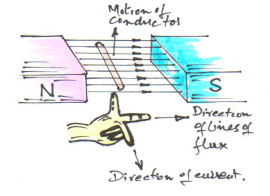Fig. 1 showing principle of electromagnetic induction & Fleming's LH Rule.

Constructionally, there is no basic difference between a dc generator and a dc motor. In fact, the same dc machine can be used interchangeably as a generator or a motor. Just like generators, dc motors are shunt wound or series wound or compound wound.

In a multi-polar dc motor Fig. 2, when its field magnets are excited and its armature conductors are supplied with current from the supply mains, they experience a force, akin to driving torque, which sets the armature rotating.

The function of commutator in the motor is the same as in a generator. It helps to develop a continuous and unidirectional torque by reversing current in each conductor as it passes through from one pole to another.

Practical dc motor consists of Magnetic frame or yoke, field poles and pole shoes, field winding comprising pole coils or field coils, armature core, armature windings or conductors, commutator, brushes and bearings.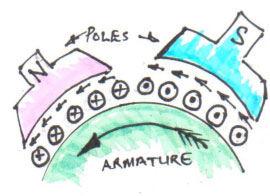Fig. 2 showing part of a multi-polar motor.

Of these, the yoke, the pole cores, armature core and air gap between the poles and the armature core form the magnetic circuit whereas the rest form the electrical circuit.

#### SIGNIFICANCE OF THE BACK E.M.F.:

When the motor armature rotates, the conductors also rotate and hence cut the flux (magnetic line of force). In accordance with the laws of electromagnetic induction, e.m.f. is induced in them, whose direction is in opposition to applied voltage. Because of its opposing direction, it is referred to as back e.m.f, Eb. Figure 3 below gives the equivalent circuit of the motor.Fig. 3 showing equivalent circuit.

The rotating armature generating back e.m.f. is like a battery of e.m.f. Eb put across supply of mains of V volts. Obviously voltage V has to drive Ia against the opposition of Eb. The power required to overcome this opposition is Eb Ia. This is the power which is converted into mechanical energy.

As can be seen from the equivalent circuit (Fig. 3) Ia = Net voltage/Resistance = (V – Eb)/Ra, where Ra is the resistance of the armature circuit.

Also Eb = (ΦZN/60) x (P/A) x 10 -8 V, where

• Φ = Flux/pole in lines
• Z = Total number of armature conductors
• N = Armature rotation speed in rpm
• P = No. of poles
• A = No. of parallel paths in armature

Normally, for wave-wound armature, A = 2 and for lap-wound armature A = P i.e. the same as number of poles.

Back e.m.f. depends, besides other factors, upon armature speed. If speed is high, Eb is large, hence armature current is small. If speed is less, then Eb is less, hence more current flows which develops more torque. So, Eb acts like a governor, in that, it makes a motor self-regulating so that it draws as much current as is necessary.

#### VOLTAGE EQUATION:

The voltage V applied across the motor armature has to overcome

a) back e.m.f. Eb!

b) armature ohmic drop IaRa

Therefore, V = Eb + IaRa – This is called the voltage equation.

If we multiply both sides of the equation by Ia, we get

V Ia = Eb Ia + Ia2Ra – This indicates that

V Ia = Electrical input to the armature

Eb Ia = Electrical equivalent of mechanical power Pm developed in the armature

Ia2Ra = Copper loss in the armature.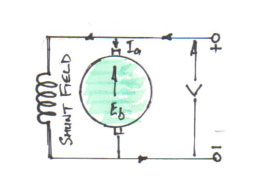Fig. 4 showing shunt field

#### CONDITION FOR MAXIMUM POWER:

Re-arranging equation V Ia = Eb Ia + Ia2Ra in terms of mechanical power, we get

Pm = V Ia – Ia2Ra

Differentiating both sides with respect Ia and equating to zero, we get dPm / d Ia = V – 2 IaRa = 0, from which we get IaRa = V/2 and as V = Eb x IaRa and IaRa = V/2 Therefore Eb = V/2.

Thus mechanical power developed by a motor is maximum when back e.m.f. is equal to half the applied voltage. This condition is not realised in practice, because in that case current would be much beyond the normal current of the motor.

#### ARMATURE TORQUE OF A MOTOR:

If T is the torque in lb-ft developed by the armature of a motor running at N rpm, then

Work done/min. = T x 2πN ft-lbs

Therefore Power in HP = (T x 2πN/33000)

We know electrical equivalent of mechanical power in the armature = Eb Ia Watts.

Therefore HP developed = Eb Ia /746

Hence we have (T x 2πN/33000) = Eb Ia /746, but

Eb = (ΦZN/60) x (P/A) x 10 -8 V

Therefore, (T x 2πN/33000) = [(ΦZN/60) x (P/A) x 10 -8] x (Ia /746)

Therefore, T = [(33000 x 10 -8) / (2π60 x 746) x (ΦZ Ia) P/A]

Therefore, T = [0.118 x 10 -8 x ΦZ Ia (P/A)] lb-ft

In SI units torque T = [0.159 x 10 -8 x ΦZ Ia (P/A)] Nm

From above equation of torque, we have seen T ∞ Φ Ia, so that

a) in the case of series wound motors, Φ is directly proportional to Ia (before saturation) because fieldwindings carry full armature current; therefore

T ∞ Ia2.

b) in the case shunt wound motor, Φ is practically constant, hence

T ∞ Ia

Also we know (T x 2πN/33000) = Eb Ia /746.

Therefore T = [(33000/2π x 746) x (Eb Ia / N)]

Therefore, T = 7.04 x (Eb Ia / N) lb-ft

#### SHAFT TORQUE, Tsh:

The whole of the armature torque, as calculated above, is not available for doing useful work, because certain percentage of it is required for supplying iron and friction losses.

The torque which is available for doing useful work is known as shaft torque Tsh and the power corresponding this torque is called brake horse power (BHP). Both of these quantities are related by formula:

BHP = Tsh x 2πN/33000 or

Tsh = 33000 x BHP/2πN lb-ft = 5252 x BHP/N lb-ft or

In terms of SI units,

Tsh = 9550 x kW/N Nm

#### Note 1HP = 746Watts.

The difference, T – Tsh, is known as lost torque.

Therefore lost torque, T – Tsh = 7.04 x (Iron & Friction Losses / N)

#### SPEED OF A DC MOTOR:

From the voltage equation, V = Eb + IaRa, we have Eb = V – IaRa

Also Eb = (ΦZN/60) x (P/A) x 10 -8 V,

Therefore, (ΦZN/60) x (P/A) x 10 -8 = V – IaRa

Therefore N = (V – IaRa/Φ) x (60A/PZ) x 108 rpm, since Eb = V – IaRa,

Therefore N = (Eb/Φ) x (60A/PZ) x 108 rpm or

N = k Eb/Φ

It shows that speed is directly proportional to back e.m.f. Eb and inversely proportional to the flux Φ.

#### For Series Motor

Let in the 1st case speed = N1, Ia1 = armature current and Φ1 = flux/pole and

Let in the 2nd case speed = N2, Ia2 = armature current and Φ2 = flux/pole, then

Using the relations whereby

N1 ∞ Eb1/ Φ1, where Eb1 = V – Ia1Ra

N2 ∞ Eb2/ Φ2, where Eb2 = V – Ia2Ra

Diving 2nd by 1st we get

N2/ N1 = (Eb2 x Φ1)/ (Eb1 x Φ2)

Prior to saturation of magnetic poles, since Φ ∞ Ia, we have

N2/ N1 = (Eb2 x Ia1)/ (Eb1 x Ia2)

#### For Shunt Motor

In this case the same equation applies i.e.

N2/ N1 = (Eb2 x Φ1)/ (Eb1 x Φ2) but if Φ2 = Φ1, then

N2/ N1 = Eb2 / Eb1

#### SPEED REGULATION:

The term speed regulation refers to the change in speed of a motor with change in applied load torque, other conditions remaining constant. By change in speed here means the change which occurs under these conditions due to inherent properties of the motor itself and not those changes which are affected through manipulation of rheostats or other speed controlling devices.

The speed regulation is defined as the change in speed when the load on the motor is reduced from rated load to zero, expressed a percent of the rated speed.

Therefore,

% speed regulation = (No Load Speed – Full Load Speed / Full Load Speed) x 100.

#### MOTOR CHARACTERISTICS:

The characteristic curves of a motor are those characteristics which show relationship between the following quantities:

a) Torque and armature current i.e. T / Iacharacteristic, also known as electrical characteristic.

b) Speed and armature current i.e. N / Ia characteristic.

c) Speed and torque i.e. N / T characteristic, also known as mechanical characteristic.

While discussing characteristics, following relationships should be remembered

T ∞ Φ Ia and N ∞ Eb/Φ.

#### Characteristics of Series Motor: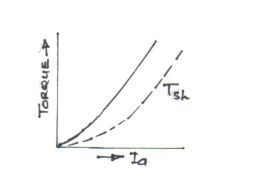Fig. 5 showing Torque (T) v Armature Current (Ia) characteristic.

#### a)T / Ia characteristic:

We have seen T ∞ Φ Ia. In this case, as field winding also carry the armature current, hence Φ ∞ Ia prior to magnetic saturation. Hence before saturation,

As T ∞ Φ Ia & Φ ∞ Ia, therefore T ∞ Ia2.

At light loads, Ia and hence ∞ is small. But as Ia increases, T increases as the square of the current. Hence T / Ia is a parabola as shown in the figure 5. After saturation, ∞ is almost independent of Ia, hence T ∞ Ia only. So, characteristics become a straight line. The shaft torque Tsh is less than armature torque due to stray losses. It is shown as dotted line in the figure.

So, the conclusion is that prior to magnetic saturation on heavy load, a series motor exerts a torque proportional to square of the armature current. Hence, where huge starting torque is required for accelerating heavy masses as in crane hoist, lifts, electric trains etc., series motors are used.

#### b) N / Ia characteristic:Fig. 6 showing Speed (N) v Armature Current (Ia) characteristic.

Variations of speed can be deduced from the formula N ∞ Eb/Φ. Change in Eb for various load currents is small, so may be neglected for the time being. With increased Ia, Φ also increases. Hence, speed varies inversely as armature current as shown in the figure 6 above.

When the load is heavy, Ia is large. Hence speed is low (this decreases Eb and allows more armature current to flow). But when load and hence Ia falls to a small value, speed becomes dangerously high. Hence a series motor should never be started without some mechanical load on it.

#### c) N / T Characteristic: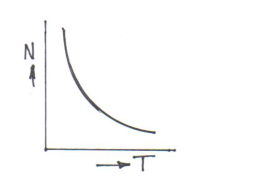Fig. 7 showing Speed (N) v Torque (T) characteristic.

The relationship between the two is as per figure 7 above. When the speed is high, torque is small and vice versa.

#### a) T / Iacharacteristic: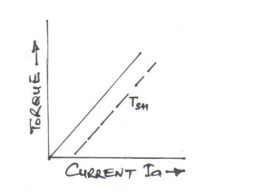Fig. 8 showing Torque (T) v Armature Current (Ia) characteristic.

Assuming ∞ to be practically constant (though at heavy loads, it decreases somewhat due to increased armature reaction), we find T ∞ Φ. Hence electrical characteristic, as shown in the figure 8, is practically a straight line through the origin. Shaft torque is shown dotted. Since heavy starting load will need high starting current, shunt motor should not be starting on heavy load.

#### b) N / Ia characteristic:

If Φ is assumed to be constant, then N would be directly proportional to Eb. As Eb is practically constant, then speed, N, would be constant as well as shown in the figure 9 below.Fig. 9 showing Speed (N) v Armature Current (Ia) characteristic.

In actual fact, both Eb & Φ decrease with increasing load and hence there is some decrease in the speed, resulting in slight droop in the curve, as shown by the dotted line. But for intense and purposes, shunt motor is taken as a constant speed motor and because of that, it is used on applications where there is sudden change in the load conditions, such as machine tools, wood-working machinery etc.

#### c) N / T characteristic:

As N is directly proportional to T, the characteristic is a drooping straight line as shown in the figure 10 below.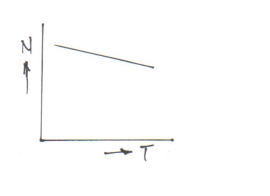Fig 10 showing Speed (N) v Torque (T) characteristic.

#### CUMULATIVE COMPOUND MOTORS:

In these motors, series excitation helps shunt excitation. The mechanical characteristics of such machines lie in between those of shunt and series motors as shown in the figure below.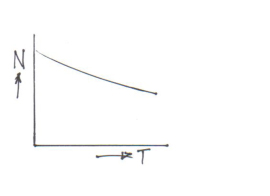Fig 11 showing Speed (N) v Torque (T) characteristic of CC motor.

Such motors are used in some coal cutting machines or for driving heavy machine tools.

#### PERFORMANCE CURVES:

a) Typical performance curve of a shunt motor (Fig. 12): It can be seen that shunt motor has definite no-load speed; hence it does run not away when load is taken off provided the field circuit remains closed. The drop in the speed from no load to full load is small. The efficiency curve is of the same shape as all electric motors and generators. The torque is directly proportional to the armature current i.e. if twice full load torque is required at starting, then shunt motor draws twice the full load current.Fig. 12 Performance curves of a Shunt-wound Motor.

b) Typical performance curve of series motor:Fig. 13 Performance curves of a Series-wound Motor.

It can be seen that drop in speed with increased load is much more prominent in series motor. Hence series motor is not suitable for applications requiring constant speed. But for a given current input, the starting torque developed by a series motor is much greater. Hence series motors are used where huge starting torques are necessary e.g. trams, cranes hoists, traction systems in railways etc. Another feature which is useful in traction work is that when a load comes on a series motor, it responds by decreasing its speed and supplies increased torque with a small increase in current.

#### SUMMARY OF APPLICATIONS OF BRUSHED DC MOTORS:

Table 1 Indicating some of the applications of DC Motors.
Type of Motor Characteristic Application
Shunt Approximately constant speed, which is adjustable, but medium stating torque. Machine tools, lathes, positive displacement pumps and blowers, compressors, reciprocating pumps etc. In fact, wherever constant speed is desirable.
Series Variable speed, which is adjustable. High starting torque. Traction work i.e. electric locomotives, rapid transit systems, trams etc. Crane hoists, conveyors etc.
Cumulative Compound Variable speed, which is adjustable. High starting torque. Intermittent high torque loads, shears and punches, elevators, conveyors, heavy planers, rolling mills, crushers etc.

#### LOSSES AND EFFICIENCY:

The losses taking place in motor are (i) Copper losses (ii) Magnetic losses and (iii) Mechanical losses.

The condition for maximum power developed by the motor is

IaRa = V/2 = Eb.

The condition for maximum efficiency is that armature copper losses are equal to constant losses – iron plus friction and windage losses.

The various stages of energy transformation in a motor and also the various losses occurring in it are given in the table below:

Table 2
A B C D E
Motor Input(VI Watts) Armature copper losses Driving power in armature (EbIa Watts) Iron + Friction & windage losses Output BHP x 746 Watts

From the table:

Overall or commercial efficiency ηc = [(E/A) x 100] %

Electrical efficiency ηe = [(C/A) x 100] %

Mechanical efficiency ηm = [(E/C) x 100] %

Note

The above write-up only describes some of the prominent brushed dc motors. Of course, there are other types such as differential compound, permanent magnet – with and without brushes and separately excited (Sepex) motors, which could add to the coverage along with a chapter on starting and speed control.

Because of the onslaught of the ac technology, people have been predicting the demise of dc motors for a long time, but they are still here, going strong and some niche applications are irreplaceable.

Param Virdi/LS (UK) LTD/ 23.02.11